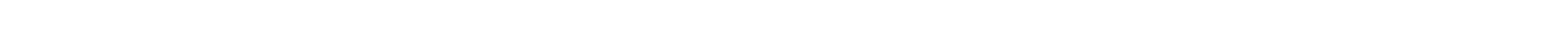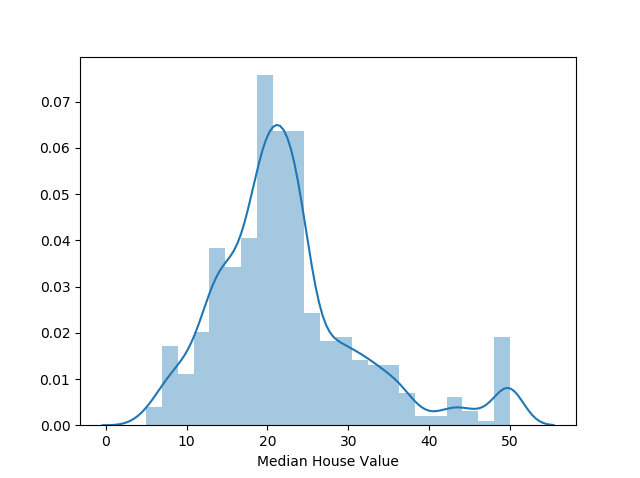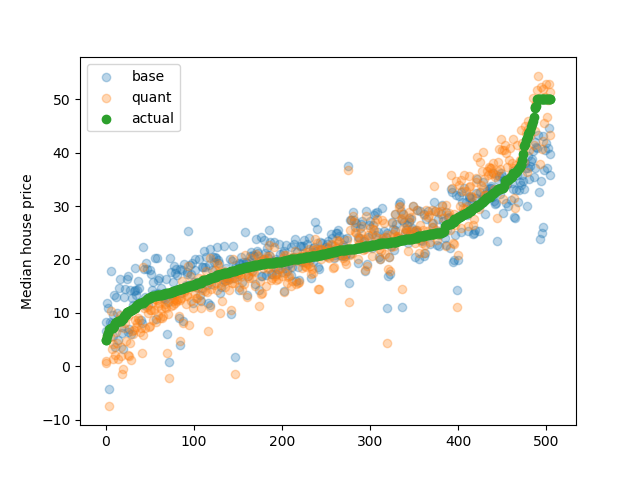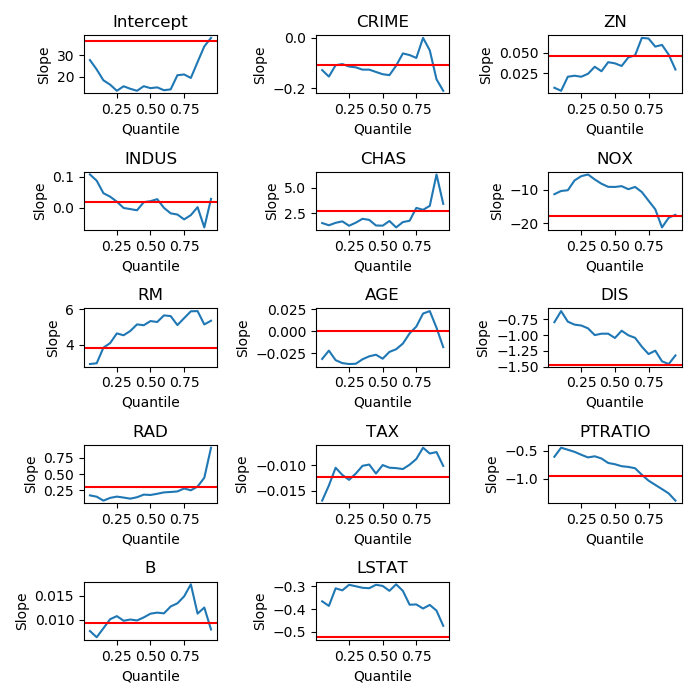Matthew Burke

Black Box Machine Learning

The trend in machine learning these days seems to be heading more and more towards deep learning and extensive tree-based ensemble algorithms, which in turn results in a lot of models becoming black box algorithms, which nobody can fully explain. In the past, researchers were forced to use more simple, explainable techniques due to lack of compute power, but now with the advent of cloud computing and the ability to spin up clusters of high-powered CPUs and GPUs, those limitations have been removed and people have to trust that their cross-validation ensures applicability to unseen data.

My current job places a lot of emphasis on interpretability of models that get put into production, eliminating that inherent need to rely on algorithms or using methods to explain local behavior of models at specific points such as LIME. This has caused me to approach problem solving in a different way and look for methods that tow the line between performance and interpretability.

Quantile Regression

Quantile regression is one of those techniques my colleagues and I have looked into that fulfills these requirements by fitting multiple linear regressions locally at different quantile points in the data.

This method captures the non-linear relationships between the covariates and the response variable close to each quantile while still having linear coefficients that are easily understandable from a human researcher for any given point. This has the potential to increase model performance drastically, especially for problems that have normally distributed response variables, where linear models tend to perform poorly outside of the mean.

Housing Example

I used the Boston housing dataset to do a quick benchmark of standard linear regression versus quantile regression based on its non-uniform distribution of the median house price (target variable):It appears as if there is a normal distribution with some outliers at the high end that may cap the median house value at 50.

I trained a standard OLS model on the data as well as a quantile regression model made up of individual regressions performed locally at each 0.05 quantile from 0 to 1 and compared the accuracy results below:

Metric Base Regression Quantile Regression
MAE 3.271 2.95
MSE 21.895 16.257
R2 0.741 0.807

The results show a definite increase in overall error reduction as well as more variance explained by the quantile regression. Additionally, if you look at the plot below, you can see that the blue points (simple OLS model) tend to have larger errors around the upper and lower points of the target variable, which is consistent with our expectations.This is all great, but how is this interpretable if we have 19 individual models fit, each with their own sets of coefficients with intercepts, slopes and confidence intervals? One easy way would be to plot the values for each covariate independently as a function of the quantile on which they were trained to see how the relationships vary in terms of the response variable. Below is a quick example comparing the simple OLS model slopes vs each of the covariates’ quantile regression results:If you take a look at the file used to create these plots/calculations, you can look at how I have done these calculations and probably augment the charts to include upper/lower bounds as well as intercept values to get an even fuller picture of how your models are functioning, to the point where you could explain to management, your mom, unwilling friends, etc…

Summary

Although quantile regression doesn’t move past the basic linear approaches of OLS, it does allow some flexibility and can be a good compromise being stuck with the most basic techniques and being able to eek out a little extra accuracy for your models without sacrificing interpretability.

Extra Note

If you aren’t constrained to linear modeling but still would prefer a powerful model with high interpretability, I’d highly recommend checking out generalized additive models, which supports non-linear modeling of linear predictors while easily displaying relationships between variables and allowing many customizations such as monotonic constraints and smoothing parameters. Stitch Fix has a fantastic article on it, and there’s a convenient python library you might find helpful as well in getting up and running quickly.